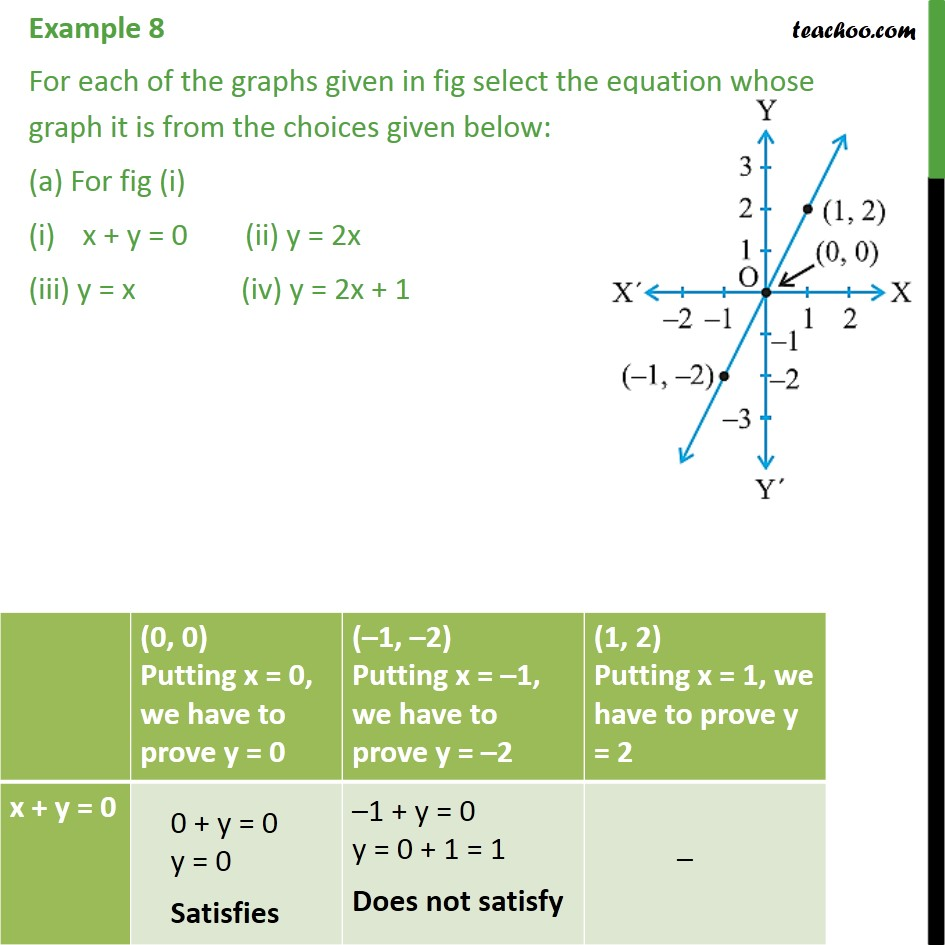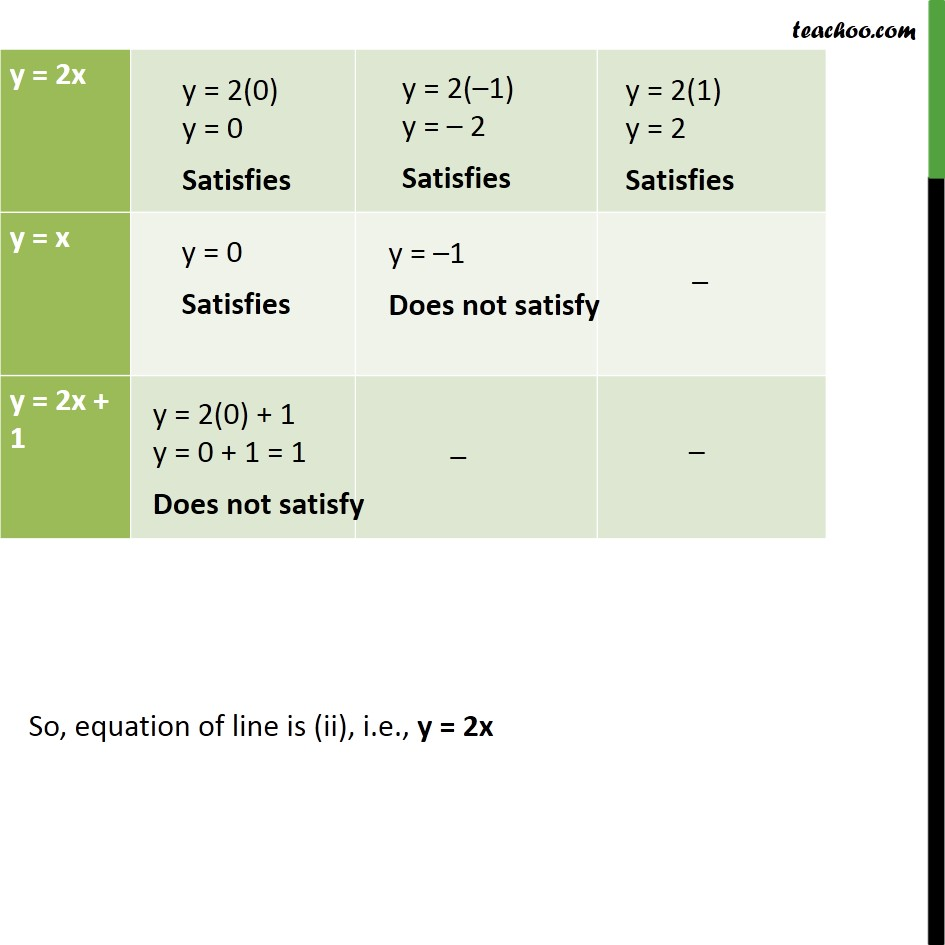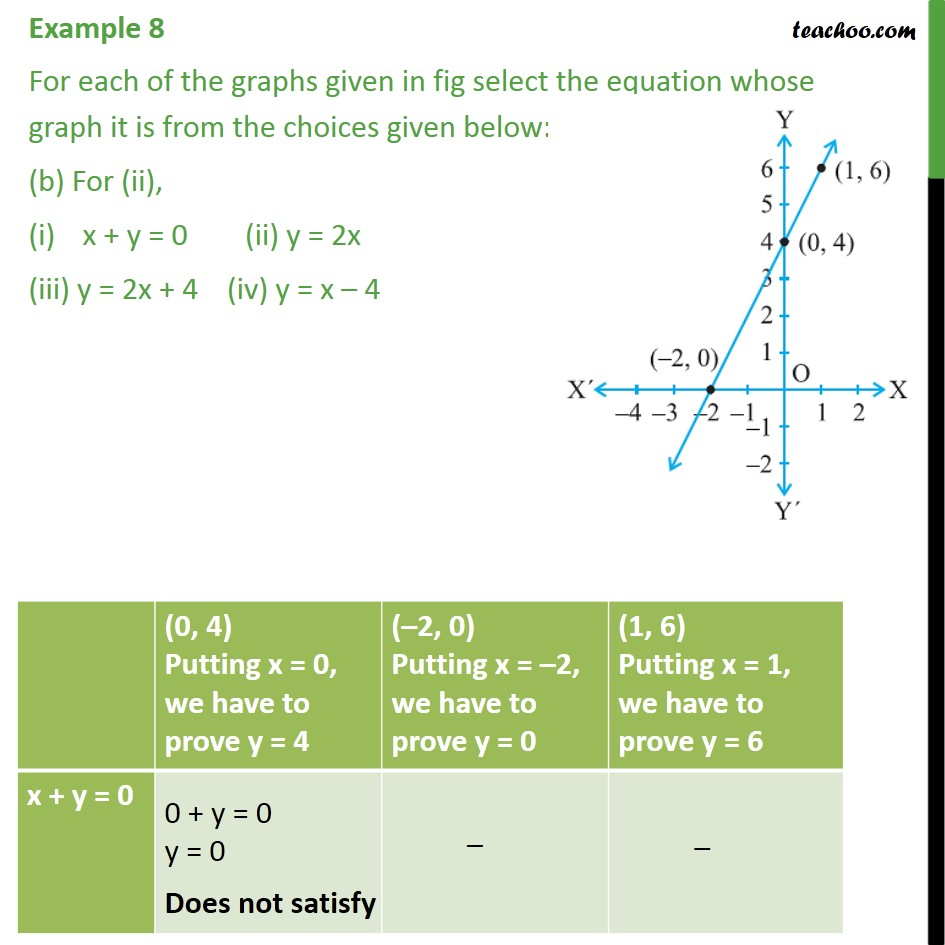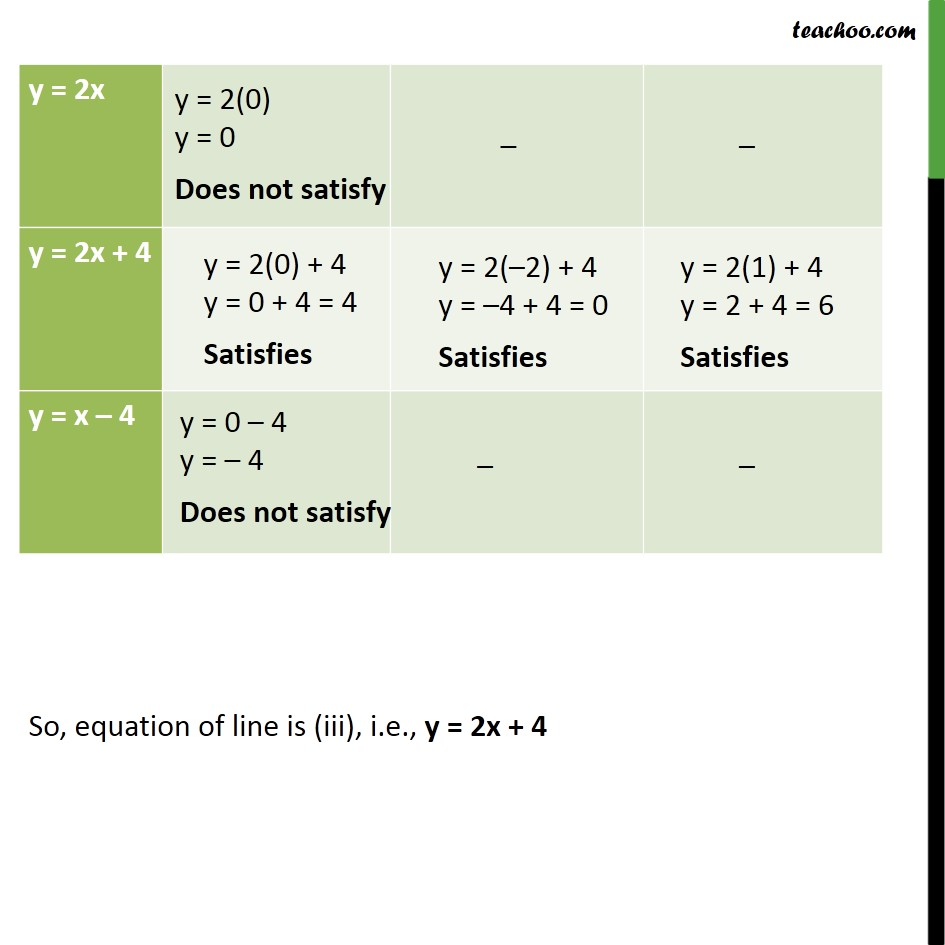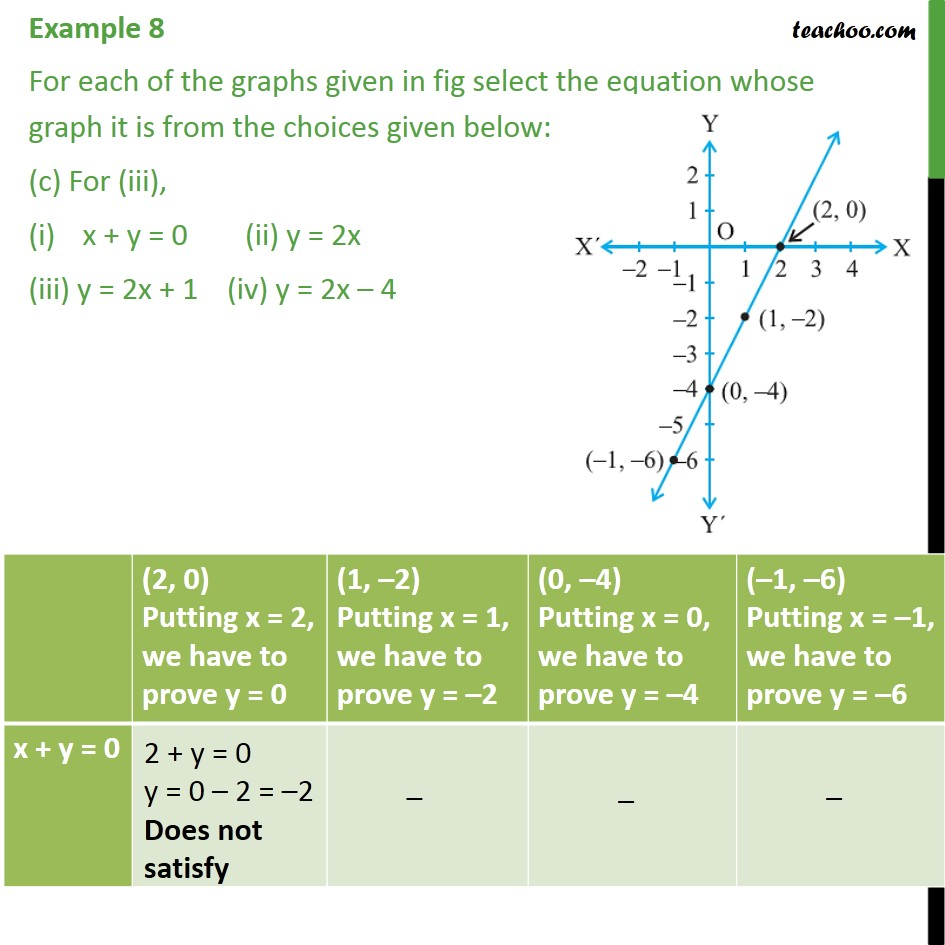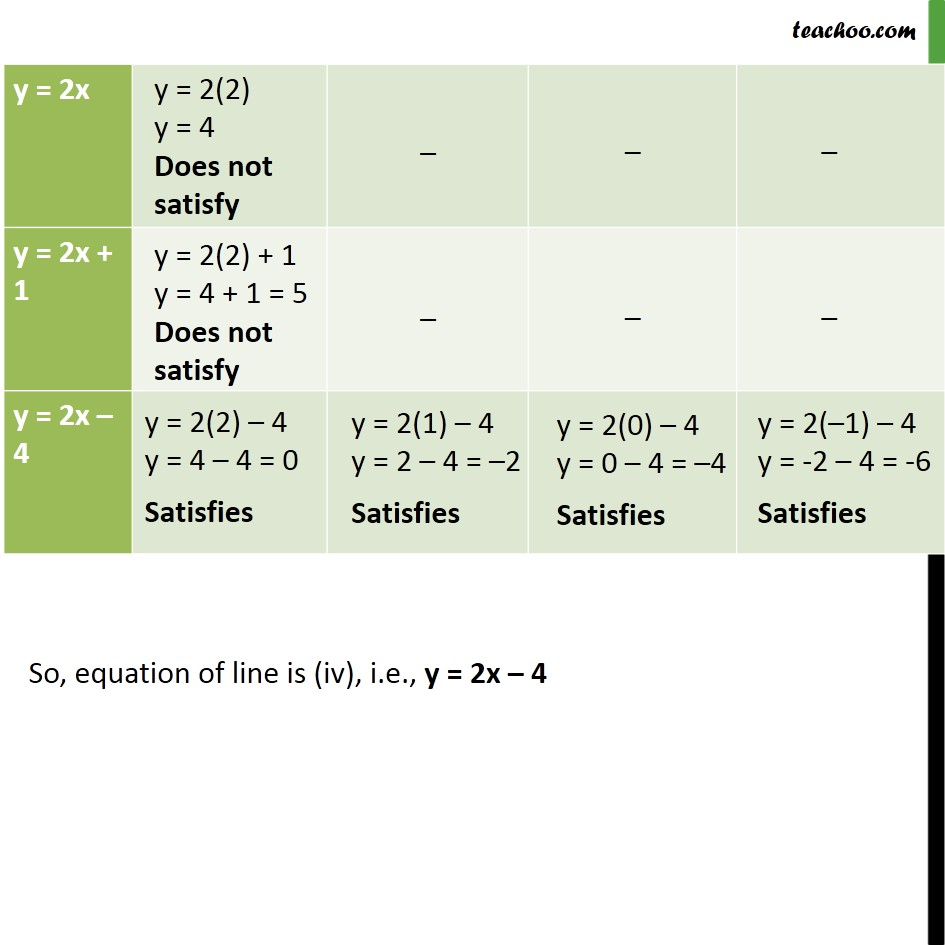1. Chapter 4 Class 9 Linear Equations in Two Variables (Term 1)
2. Concept wise
3. Graph of linear equations

Transcript

Example 8 For each of the graphs given in fig select the equation whose graph it is from the choices given below: (a) For fig (i) x + y = 0 (ii) y = 2x (iii) y = x (iv) y = 2x + 1 So, equation of line is (ii), i.e., y = 2x Example 8 For each of the graphs given in fig select the equation whose graph it is from the choices given below: (b) For (ii), x + y = 0 (ii) y = 2x (iii) y = 2x + 4 (iv) y = x 4 So, equation of line is (iii), i.e., y = 2x + 4 Example 8 For each of the graphs given in fig select the equation whose graph it is from the choices given below: (c) For (iii), x + y = 0 (ii) y = 2x (iii) y = 2x + 1 (iv) y = 2x 4 So, equation of line is (iv), i.e., y = 2x 4

Graph of linear equations